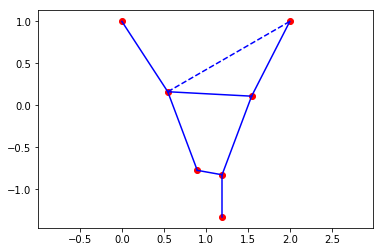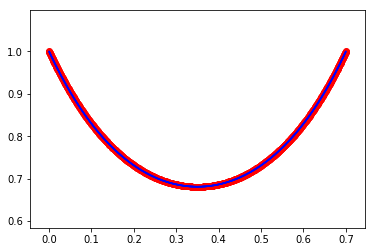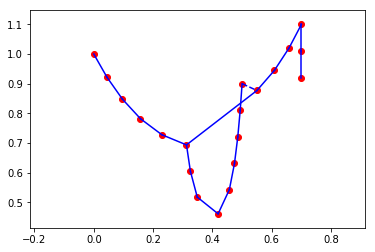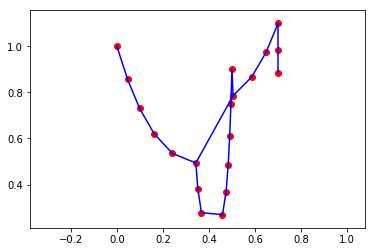## Equilibrium of a system of weights connected by strings/springs¶

In this notebook we show how to solve the following problem: Find the equlibrium of a system of masses connected by a system of strings, with some masses being assigned fixed coordinates (attached to the wall, say). See the next picture.Suppose we have $n$ masses with weights $w_1,\ldots,w_n$, and the length of the string between $i$ and $j$ is $\ell_{ij}$ for some set $L$ of pairs of indices $(i,j)$ (we assume $\ell_{ij}$ is not defined if there is no connection). The strings themselves have no mass. We also have a set $F$ of indices such that the $i$-th point is fixed to have coordinates $f_i$ if $i\in F$. The equilibrium of the system is a configuration which minimizes potential energy. With this setup we can write our problem as:

\begin{equation} \begin{array}{ll} minimize & g\cdot \sum_i w_ix_i^{(2)} \\ s.t. & \|x_i-x_j\|\leq \ell_{ij},\ ij\in L \\ & x_i = f_i,\ i\in F \end{array} \end{equation}

where $x\in (\mathbf{R}^n)^2$, $x_i^{(2)}$ denotes the second (vertical) coordinate of $x_i$ and $g$ is the gravitational constant.

Here is a sample problem description.

In :
w = [0.0, 1.1, 2.2, 0.0, 2.1, 2.2, 0.2]
l = {(0,1): 1.0, (1,2): 1.0, (2,3): 1.0, (1,4): 1.0, (4,5): 0.3, (5,2): 1.0, (5,6): 0.5, (1,3): 8.0}
f = {0: (0.0,1.0), 3: (2.0,1.0)}
g = 9.81


Now we can formulate the problem using Mosek Fusion:

In :
from mosek.fusion import *

# w - masses of points
# l - lengths of strings
# f - coordinates of fixed points
# g - gravitational constant
def stringModel(w, l, f, g):
n, m = len(w), len(l)
starts = [ lKey for lKey in l.keys() ]
ends = [ lKey for lKey in l.keys() ]

M = Model("strings")

# Coordinates of points
x = M.variable("x", [n, 2])

# A is the signed incidence matrix of points and strings
A = Matrix.sparse(m, n, list(range(m))+list(range(m)), starts+ends, [1.0]*m+[-1.0]*m)

# ||x_i-x_j|| <= l_{i,j}
c = M.constraint("c", Expr.hstack(Expr.constTerm(list(l.values())), Expr.mul(A, x)),
Domain.inQCone() )

# x_i = f_i for fixed points
for i in f:
M.constraint(x.slice([i,0], [i+1,2]), Domain.equalsTo(list(f[i])).withShape([1,2]))

# sum (g w_i x_i_2)
M.objective(ObjectiveSense.Minimize,
Expr.mul(g, Expr.dot(w, x.slice([0,1], [n,2]))))

# Solve
M.solve()
if M.getProblemStatus(SolutionType.Interior) == ProblemStatus.PrimalAndDualFeasible:
return x.level().reshape([n,2]), c.dual().reshape([m,3])
else:
return None, None


Here is a quick description of how we use vectorization to deal with all the conic constraints in one go. The matrix $A$ is the incidence matrix between the masses and the strings, with coefficients $+1, -1$ for the two endpoints of each string. It is chosen so that the product $Ax$ has rows of the form

$$(x_i^{(1)} - x_j^{(1)}, x_i^{(2)} - x_j^{(2)})$$

for all pairs $i,j$ for which $\ell_{ij}$ is bounded. Stacking the values of $\ell$ in the left column produces a matrix with each row of the form

$$(\ell_{ij}, x_i^{(1)} - x_j^{(1)}, x_i^{(2)} - x_j^{(2)})$$

and a conic constraint is imposed on all the rows, as required.

The objective and linear constraints show examples of slicing the variable $x$.

The function returns the coordinates of the masses and the values of the dual conic variables. A zero dual value indicates that a particular string is hanging loose, and a nonzero value means it is fully stretched.

All we need now is to define a display function and we can look at some plots.

In :
%matplotlib inline
# x - coordinates of the points
# c - dual values of string length constraints
# d - pairs of points to connect
def display(x, c, d):
import matplotlib.pyplot as plt
fig, ax = plt.subplots()
# Plot points
ax.scatter(x[:,0], x[:,1], color="r")
# Plot fully stretched strings (nonzero dual value) as solid lines, else dotted lines
for i in range(len(c)):
col = "b" if c[i] > 1e-4 else "b--"
ax.plot([x[d[i]], x[d[i]]], [x[d[i]], x[d[i]]], col)
ax.axis("equal")
plt.show()

In :
x,c = stringModel(w, l, f, g)

if x is not None:
display(x, c, list(l.keys()))How about we find a discrete approximation to the catenary:

In :
n = 1000
w = [1.0]*n
l = {(i,i+1): 1.0/n for i in range(n-1)}
f = {0: (0.0,1.0), n-1: (0.7,1.0)}
g = 9.81

x,c = stringModel(w, l, f, g)
if x is not None:
display(x, c, list(l.keys()))We can also have more suspension points and more complicated shapes:

In :
n = 20
w = [1.0]*n
l = {(i,i+1): 0.09 for i in range(n-1)}
l.update({(5,14): 0.3})
f = {0: (0.0,1.0), 13: (0.5,0.9), 17: (0.7,1.1)}
g = 9.81

x,c = stringModel(w, l, f, g)
if x is not None:
display(x, c, list(l.keys()))## Duality and feasibility¶

The dual problem is as follows:

\begin{equation} \begin{array}{ll} maximize & -\sum_{ij\in L}\ell_{ij}y_{ij} - \sum_{i\in F}f_i\circ z_i\\ s.t. & y_{ij}\geq \|v_{ij}\|,\ ij\in L \\ & \sum_{j~:~ij\in L} v_{ij}\mathrm{sgn}_{ij} + \left(\begin{array}{c}0\\ gw_i\end{array}\right) +z_i = 0, \ i=1,\ldots,n \end{array} \end{equation}

where $\mathrm{sgn}_{ij}=+1$ if $i>j$ and $-1$ otherwise and $\circ$ is the dot product. The variables are $(y_{ij},v_{ij})\in \mathbf{R}\times\mathbf{R}^2$ for $ij\in L$ and $z_i\in\mathbf{R}^2$ for $i\in F$ (we assume $z_i=0$ for $i\not\in F$).

Obviously (!) the linear constraints describe the equilibrium of forces at every mass. The ingredients are: the vectors of forces applied through adjacent strings ($v_{ij}$), gravity, and the attaching force holding a fixed point in its position. By proper use of vectorization this is much easier to express in Fusion than it looks:

In :
def dualStringModel(w, l, f, g):
n, m = len(w), len(l)
starts = [ lKey for lKey in l.keys() ]
ends = [ lKey for lKey in l.keys() ]

M = Model("dual strings")

x = M.variable(Domain.inQCone(m,3))       #(y,v)
y = x.slice([0,0],[m,1])
v = x.slice([0,1],[m,3])
z = M.variable([n,2])

# z_i = 0 if i is not fixed
for i in range(n):
if i not in f:
M.constraint(z.slice([i,0], [i+1,2]), Domain.equalsTo(0.0))

B = Matrix.sparse(m, n, list(range(m))+list(range(m)), starts+ends, [1.0]*m+[-1.0]*m).transpose()
w2 = Matrix.sparse(n, 2, range(n), *n, [-wT*g for wT in w])

# sum(v_ij *sgn(ij)) + z_i = -(0, gw_i) for all vertices i
M.constraint(Expr.add( Expr.mul(B, v), z ), Domain.equalsTo(w2))

# Objective -l*y -fM*z
fM = Matrix.sparse(n, 2, list(f.keys())+list(f.keys()), *len(f)+*len(f),
[pt for pt in f.values()] + [pt for pt in f.values()])

M.solve()


Let us quickly discuss the possible situations regarding feasibility:

• The system has an equilibrium --- the problem is primal feasible and dual feasible.
• The strings are too short and it is impossible to stretch the required distance between fixed points --- the problem is primal infeasible.
• The system has a component that is not connected to any fixed point, hence some masses can keep falling down indefinitely, causing the problem primal unbounded. Clearly the forces within such component cannot be balanced, so the problem is dual infeasible.

## Springs¶

We can extend this to consider infinitely strechable springs instead of fixed-length strings connecting the masses. The next model appears in Applications of SOCP by Lobo, Boyd, Vandenberghe, Lebret. We will now interpret $\ell_{ij}$ as the base length of the spring and assume that the elastic potential energy stored in the spring at length $x$ is

$$E_{ij}=\left\{\begin{array}{ll}0 & x\leq \ell_{ij}\\ \frac{k}{2}(x-\ell_{ij})^2 & x>\ell_{ij}\end{array}\right.$$

That leads us to consider the following second order cone program minimizing the total potential energy:

\begin{equation} \begin{array}{ll} minimize & g\cdot \sum_i w_ix_i^{(2)} + \frac{k}{2}\sum_{ij\in L} t_{ij}^2 \\ s.t. & \|x_i-x_j\|\leq \ell_{ij}+t_{ij},\ ij\in L \\ & 0\leq t_{ij},\ ij\in L \\ & x_i = f_i,\ i\in F \end{array} \end{equation}

If $t$ denotes the vector of $t_{ij}$ then using a rotated quadratic cone for $(1,T,t)$:

$$2\cdot 1\cdot T\geq \|t\|^2$$

will place a bound on $\frac12\sum t_{ij}^2$. We now have a simple extension of the first model.

In :
# w - masses of points
# l - lengths of strings
# f - coordinates of fixed points
# g - gravitational constant
# k - stiffness coefficient
def elasticModel(w, l, f, g, k):
n, m = len(w), len(l)
starts = [ lKey for lKey in l.keys() ]
ends = [ lKey for lKey in l.keys() ]

M = Model("strings")
x = M.variable("x", [n, 2])                 # Coordinates
t = M.variable(m, Domain.greaterThan(0.0))  # Streching

T = M.variable(1)                           # Upper bound
M.constraint(Expr.vstack(T, Expr.constTerm(1.0), t), Domain.inRotatedQCone())

# A is the signed incidence matrix of points and strings
A = Matrix.sparse(m, n, list(range(m))+list(range(m)), starts+ends, [1.0]*m+[-1.0]*m)

# ||x_i-x_j|| <= l_{i,j} + t_{i,j}
c = M.constraint("c", Expr.hstack(Expr.add(t, Expr.constTerm(list(l.values()))), Expr.mul(A, x)),
Domain.inQCone() )

# x_i = f_i for fixed points
for i in f:
M.constraint(x.slice([i,0], [i+1,2]), Domain.equalsTo(list(f[i])).withShape([1,2]))

# sum (g w_i x_i_2) + k*T
M.objective(ObjectiveSense.Minimize,

# Solve
M.solve()
if M.getProblemStatus(SolutionType.Interior) == ProblemStatus.PrimalAndDualFeasible:
return x.level().reshape([n,2]), c.dual().reshape([m,3])
else:
return None, None

In :
n = 20
w = [1.0]*n
l = {(i,i+1): 0.09 for i in range(n-1)}
l.update({(5,14): 0.3})
f = {0: (0.0,1.0), 13: (0.5,0.9), 17: (0.7,1.1)}
g = 9.81
k = 800

x, c = elasticModel(w, l, f, g, k)
if x is not None:
display(x, c, list(l.keys()))This work is licensed under a Creative Commons Attribution 4.0 International License. The MOSEK logo and name are trademarks of Mosek ApS. The code is provided as-is. Compatibility with future release of MOSEK or the Fusion API are not guaranteed. For more information contact our support.